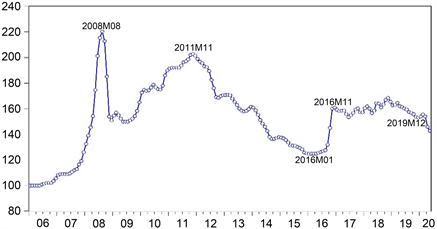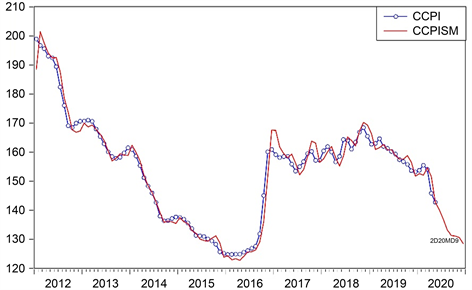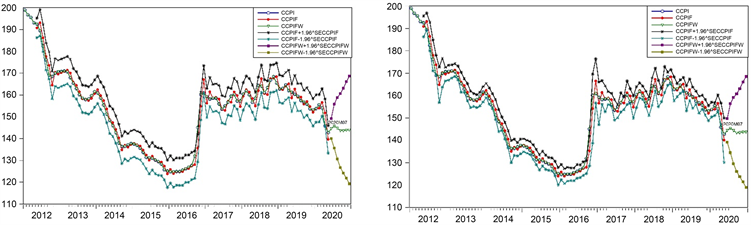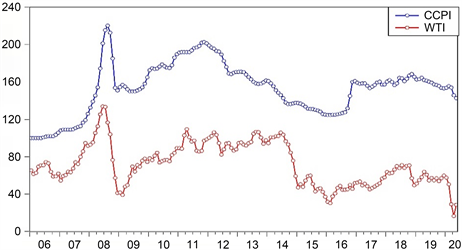﻿ 基于时间序列分析的我国煤炭价格指数研究

# 基于时间序列分析的我国煤炭价格指数研究Research on China Coal Price Index Based on Time Series Analysis

Abstract: In this paper, China coal price index (CCPI) issued by China Coal Industry Association is selected as the research object of coal price. Based on the exponential smoothing method, ARIMA-ARCH model and cointegration-error correction model of time series analysis, this paper makes a systematic study of China coal price index. The research shows that the cyclical performance of China coal price index is different before and after 2012; It can be predicted that China’s coal price will continue to decline in 2020; China coal price is strongly, persistent and asymmetric when it is influenced by external events or policies, and the impact of good news and bad news on price volatility is equal; the current fluctuation of International WTI crude oil price will have a significant impact on the current fluctuation of China coal price index, and the error of the previous period will have a signif-icant impact on the current fluctuation. The impact of volatility is also highly significant. In the fu-ture, the China coal price index can be further studied by using more frequent data, dividing coal prices into different periods, and from a global perspective.

1. 引言

2006年开始我国煤炭价格全面开放，由市场进行定价，2012年开始煤炭供给侧改革，煤炭行业经济效益显著提高。本文以2006年1月开始的中国煤炭价格指数为研究对象，对2012年1月以后的中国煤炭价格指数进行着重分析。

2. 描述性统计Figure 1. Time series chart of CCPIFigure 2. Seasonal factors of CCPIFigure 3. Exponential smoothing forecast result of CCPI

3. 基于ARIMA-ARCH模型的研究

1) 时序图、自相关图和单位根检验都显示该序列为非平稳序列，对原时序进行一阶差分处理后，经过单位根及白噪声检验，对应时序为平稳白噪声序列，即原时序为1阶单整非纯随机时序。

2) 对差分后时序进行ARMA模型建模，根据样本自相关和偏自相关系数进行模型定阶，结合AIC、SBC信息准则，最终选定ARIMA(0,1,2)模型，拟合结果如下：

${y}_{t}=\left(1-0.94B-0.26{B}^{2}\right){\epsilon }_{t},{\sigma }_{\epsilon }^{2}=5.10$.

3) 基于ARCH-LM检验、ARCH-QLB检验和残差正态性检验对上述中两个均值方程的残差平方进行ARCH效应检验，结果显示残差序列都具有显著条件异方差性，经过一系列尝试，最终对ARIMA(3,1,0)模型残差序列拟合ARCH(1)-正态分布模型，拟合结果如下：

${a}_{t}={e}_{t}\sqrt{{h}_{t}},{e}_{t}\stackrel{iid}{~}N\left(0,1\right),\text{\hspace{0.17em}}\text{\hspace{0.17em}}\text{\hspace{0.17em}}\text{\hspace{0.17em}}\text{\hspace{0.17em}}\left\{\begin{array}{l}{y}_{t}=0.87{y}_{t-1}-0.59{y}_{t-2}+0.28{y}_{t-3}+{a}_{t},\\ {h}_{t}=E\left({\epsilon }_{t}^{2}\right)=2.11+{0.28}_{t-1}^{2}.\end{array}$

4) 经过ARCH-QLB检验，ARCH(1)模型标准化残差平方不再存在ARCH效应，说明我国煤炭价格指数序列均值方程加入ARCH模型后，消除了残差序列中存在的自回归条件异方差性，ARCH(1)模型已将残差序列平方的相关性提取完全。Figure 4. Forecast result of ARIMA model (left) and ARCH model (right)

4. 基于协整–误差修正模型的研究Figure 5. Time series chart of CCPI and WTI

1) 通过图5可确定我国煤炭价格指数 ${y}_{t}$ 与WTI原油价格 ${x}_{t}$ 存在明显的同变关系，接下来对两者之间协整关系的存在性进行检验，并进一步构建误差修正模型。

2) 通过单位根检验可知我国煤炭价格指数 ${y}_{t}$ 与WTI原油价格 ${x}_{t}$ 皆为1阶单整非白噪声序列。结果见表1表2。构造我国煤炭价格指数序列 ${y}_{t}$ 与WTI原油价格序列 ${w}_{t}$ 的回归模型：

${y}_{t}=111.47+0.59{w}_{t}+{\epsilon }_{t}$

3) 对以上模型残差序列进行单位根检验，检验结果见表3：可判定残差具有平稳性，进而我国煤炭价格指数序列 ${y}_{t}$ 与WTI原油价格序列 ${w}_{t}$ 之间的协整关系成立。Table 1. Correlogram of d (WTI)Table 2. Augmented dickey-fuller unit root test on d (WTI)Table 3. Augmented dickey-fuller unit root test on model residuals

4) 协整关系的确立，说明我国煤炭价格指数与WTI原油价格之间存在着长期稳定的均衡关系，这是从整体上来说，但在时序观测的局部时间段内，可能会在局部时间段内单整时序之间距离的比较近或比较远，不再同步，意味着这种均衡关系有可能被打破。为了测度这种局部不均衡状态，进一步构建误差修正模型：

$\nabla {y}_{t}=0.28\nabla {x}_{t}-0.04EC{M}_{t-1}+{\epsilon }_{t}$，其中 $EC{M}_{t-1}={y}_{t-1}-111.47-0.59{x}_{t}$

5. 总结

1) 基于指数平滑方法，可以发现我国煤炭价格指数周期性的表现在2012年前后是不相同的，且最近7年周期性趋于稳定。运用Holt-Winters三参数乘法指数平滑法，可以预测2020年我国煤炭价格持续走低。

2) 基于ARIMA-ARCH模型结果，ARCH系统通过了显著性检验，说明均值方程的残差平方确实存在条件异方差性；ARCH模型相对于均值模型，AIC，SC都显著减小，说明ARCH模型较均值方程对应模型对我国煤炭价格指数时序的拟合效果更好，考虑条件异方差的模型预测结果明显比不考虑异方差的模型预测结果更为精确。两个预测结果均显示，2020年我国煤炭价格最高值将出现在7月。ARCH系数大于GARCH系数说明我国煤炭价格受外在事件或政策影响反应强烈，但对系统内部造成的影响可忽略，且不存在非对称效应，好消息和坏消息对波动性的冲击一样大。

3) 基于协整–误差修正模型结果，可以看出WTI原油价格指数当期波动对我国煤炭价格指数当期波动有显著影响，上期误差对当期波动的影响也高度显著；同时WTI原油价格指数的当期波动对我国煤炭价格指数的当期波动调整幅度很大，上期误差对当期我国煤炭价格指数的当期波动调整幅度较小。

NOTES

*通讯作者。

 殷琴. 煤炭经济运行风险评价研究[D]: [硕士学位论文]. 北京: 中国矿业大学, 2014.

 刘畅, 王旭冉. 中国煤炭价格波动与去产能政策的选择[J/OL]. 西安交通大学学报(社会科学版): 1-13.

 邹绍辉, 张甜. 基于GARCH模型的煤炭价格波动规律研究[J]. 价格月刊, 2017(9): 13-18.

 张艳芹. 金融视角的我国煤炭价格波动研究[D]: [博士学位论文]. 北京: 中国矿业大学, 2016.

 张建英. 我国煤炭价格影响因素的VAR模型分析[J]. 经济问题, 2016(1): 108-112.

 王文琦. 新常态下中国煤炭价格波动影响因素研究—基于Var模型的协整分析[J]. 经济论坛, 2016(5): 83-89.

 冯雨, 冯华敏. 我国煤炭价格指数发展历程及其展望[J]. 中国煤炭, 2019, 45(5): 10-14 + 66.

 王燕. 应用时间序列分析[M]. 北京: 中国人民出版社, 2015.

 张艳芹, 刘满芝. 我国煤炭价格波动研究—基于GARCH模型的实证分析[J]. 价格理论与实践, 2014(3): 75-76.

Top